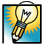Jim Worthey • Lighting & Color Research • jim@jimworthey.com • 301-977-3551 • 11 Rye Court, Gaithersburg, MD 20878-1901, USA
 Why do Color Matching Experiments use Red, Green, and Blue primaries? What wavelengths are best?

Color Matching Functions
In a visual colorimetry experiment, a human sets a mixture of 3 primaries to match a test color. For example, a narrow band Test Light has unit power (1 watt, for example), and its power is held constant while its wavelength is varied through the visible spectrum. At each setting of the test light, the observer adjusts the 3 primary lights so that their mixture matches the test light. In quasi-algebraic terms, the observer's setting can be written:

test = Rred + Ggreen + Bblue .

The words in bold face indicate narrow-band lights, not algebraic variables. Variables R, G, B will be the power settings of the so-called primary lights. For most settings of the test wavelength, one of the power settings will be a negative number. In the laboratory, if R <0, it means that red light is added to test , rather than combining with the mixture of Ggreen + Bblue . Now when test is varied through the spectrum and  R, G, B are graphed, the 3 settings are called color matching functions. The graph below shows a set of color matching functions, or cmf's. But why is the graph moving?

 Animated Color Matching Functions If you have the Flash plug-in in your browser, you should see moving graphs above. Starting with the usual 2-degree observer of the CIE, color-matching functions were calculated for 37 unique combinations of primary wavelengths. The 37 graphs are then combined to make a 75-frame animation. The diamond shapes mark the wavelengths of the primary lights. From starting values of 603, 538, and 446 nm, the primary wavelengths are varied one at a time, first decreasing, then increasing, then decreasing back to the starting value. Then the next primary is varied, and so forth. The dashed lines are set at the same wavelengths as those starting values. The numbers, 603, 538, and 446, are the Prime Colors, so named by Thornton. When the primaries are set to these wavelengths, then the primary and the peak are at the same wavelength, and the peak heights are 1.0, the lowest possible value.

 So Far, So Good. But why are the primary lights always red, green and blue? The method by which the graphs are calculated is right out of the textbooks. There is nothing new or speculative in computing the color matching functions for a set of 3 primary wavelengths. Similar methods were used in comparing data from different laboratories when the CIE 1931 system was first developed. The traditional textbook discussion teaches that alternate sets of primaries may be used, but leaves some questions unanswered. Why are the primaries always red, green, and blue? Are there 3 wavelengths that work the best? The animation, based on detailed calculations, teaches two interesting lessons: 1. The peaks of the cmf's occur at certain wavelengths that are innate, and are not particularly dependent on the choice of primaries. [If the primaries were changed sufficiently, two of them could be interchanged, upsetting this simple observation. The animation looks at a more limited perturbation of the primaries.] 2. When the primaries are set to the prime colors, 603, 538, and 446 nm, then the cmf's peak at unit power. When one primary wavelength is perturbed from its prime color, the maximum power needed in that primary increases a little or a lot. These are not new observations. They have been published by William A. Thornton and by Jozef Cohen, and a related theorem was published by Michael H. Brill. My goal here is to let you observe these facts for yourself, with the aid of computer animation. The same ideas can be re-stated in a more intuitive way. Suppose that a child asks what the color matching functions mean. Keep in mind there is only one test light, varying through the entire spectrum. Therefore, the three humps in the color matching functions show the 3 wavelength regions where the test light acts most strongly in mixtures. There are 3 humps because color is 3-dimensional, and they denote the regions where the test light acts strongly in the independent blue, green, and red directions in color space. The demo shows that the functions peak at wavelengths that change little when the primary wavelengths are perturbed. We note that "certain wavelengths act strongly in mixtures." After staring at the animation for a short time, you can make a stronger statement. There are 3 well-defined wavelengths that act most strongly in mixtures. They are a red, a green, and a blue, at 603, 538, and 446 nm. How can there be three "most important" wavelengths? That's simple. Color is a vector and the full use of color vision calls for stimulation in 3 independent dimensions. Illuminating engineers are taught that 555 nm is the one important wavelength. The lighting textbooks are wrong.web site designed by Nick Worthey